# Introduction to the biosensors.usc package

The biosensor.usc aims to provide a unified and user-friendly framework for using new distributional representations of biosensors data in different statistical modeling tasks: regression models, hypothesis testing, cluster analysis, visualization, and descriptive analysis. Distributional representations are a functional extension of compositional time-range metrics and we have used them successfully so far in modeling glucose profiles and accelerometer data. However, these functional representations can be used to represent any biosensor data such as ECG or medical imaging such as fMRI.

## Installation

You can install this package from source code using the devtools library:

devtools::install_github("glucodensities/biosensors.usc@main", type = "source")

## Quick Start

The purpose of this section is to give users a general sense of the package, including the components, what they do and some basic usage. We will briefly go over the main functions, see the basic operations and have a look at the outputs. Users may have a better idea after this section what functions are available. More details are available in the package documentation.

First, we load the biosensors.usc package:

library(biosensors.usc)

## Package example

This example is extracted from the paper: Hall, H., Perelman, D., Breschi, A., Limcaoco, P., Kellogg, R., McLaughlin, T., Snyder, M., Glucotypes reveal new patterns of glucose dysregulation, PLoS biology 16(7), 2018.

We include part of this data set in the inst/exdata folder. This data set has two different types of files. The first one contains the functional data, which csv files must have long format with, at least, the following three columns: id, time, and value, where the id identifies the individual, the time indicates the moment in which the data was captured, and the value is a monitor measure:

file1 = system.file("extdata", "data_1.csv", package = "biosensors.usc")

The second type contains the clinical variables. This csv file must contain a row per individual and must have a column id identifying this individual:

file2 = system.file("extdata", "variables_1.csv", package = "biosensors.usc")

From these files, biosensor data can be loaded as follow:

data1 = load_data(file1, file2)
class(data1)
#>  "biosensor"
names(data1)
#>  "data"      "densities" "quantiles" "variables"

The load_data function returns a biosensor object. This object contains a data frame with biosensor raw data, a functional data object (fdata) with a non-parametric density estimation, a functional data object (fdata) with the empirical quantile estimation, and a data frame with the covariates.

We also provide a way to generate biosensor data from the quantile regression model V + V2 * v + tau * V3 * Q0, where Q0 is a truncated random variable, v = 2 * X, tau = 2 * X, V ~ Unif(-1, 1), V2 ~ Unif(-1, -1), V3 ~ Unif(0.8, 1.2), and E(V|X) = tau * Q0:

another_data_example = generate_data(n=100, Qp=100, Xp=5)
head(another_data_example$variables) #> V1 V2 V3 V4 V5 #> 1 0.40124632 0.8393113 0.1416429 0.7080482 0.35399763 #> 2 0.09912248 0.4032639 0.1628153 0.1910880 0.14855589 #> 3 0.82880080 0.7071121 0.7025730 0.6263756 0.46601274 #> 4 0.58616056 0.1454937 0.7117841 0.2025261 0.04063063 #> 5 0.87658390 0.9805933 0.7003993 0.9266100 0.30451721 #> 6 0.18407365 0.6245224 0.9331051 0.3059880 0.12594252 plot(another_data_example$quantiles, main="Simulated data")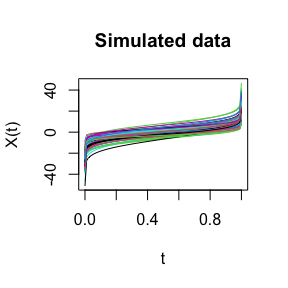### Wasserstein regression and prediction

You can call the Wasserstein regression, using as predictor the distributional representation and as response a scalar outcome. In this example, we use the previously loaded biosensor data and the BMI covariate:

regm = regmod_regression(data1, "BMI")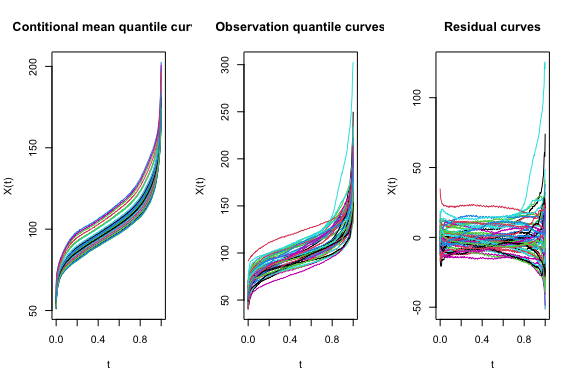As result, this function returns the fitted regression and plots the residuals of the curves against the fitted values. In addition, the function plots the confidance band of the mean values.

You can obtain the regression prediction from a kxp matrix of input values for regressors for prediction, where k is the number of points we do the prediction and p is the dimension of the input variables:

xpred = as.matrix(25)
pred = regmod_prediction(regm, xpred)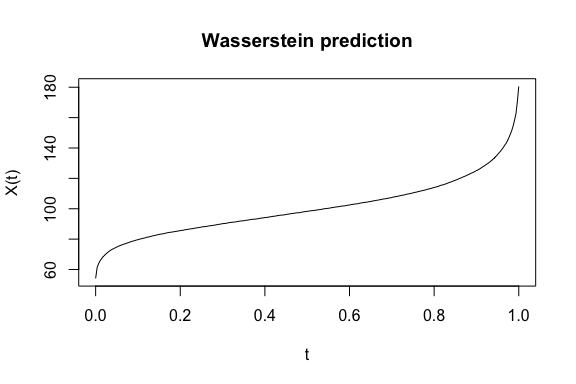### Ridge regression

Call the Ridge regression as follows, using as predictor the distributional representation and as response a scalar outcome:

ridg = ridge_regression(data1, "BMI")

Use the following function to obtain the functional non-parametric Nadaraya-Watson regression with 2-Wasserstein distance, using as predictor the distributional representation and as response a scalar outcome:

nada = nadayara_regression(data1, "BMI")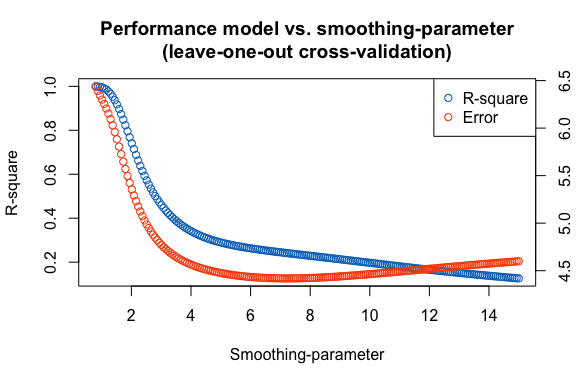Use the previously computed Nadaraya-Watson regression to obtain the regression prediction given the quantile curves:

npre = nadayara_prediction(nada, t(colMeans(data1$quantiles$data)))

### Hypothesis testing

You can perform hypothesis testing between two random samples of distributional representations to detect differences in scale and localization (ANOVA test) or distributional differences (Energy distance).

file3 = system.file("extdata", "data_2.csv", package = "biosensors.usc")
file4 = system.file("extdata", "variables_2.csv", package = "biosensors.usc")
data2 = load_data(file3, file4)

Then call the following function:

htest = hypothesis_testing(data1, data2)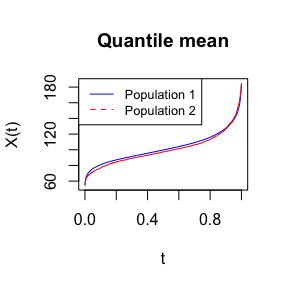#> Warning: executing %dopar% sequentially: no parallel backend registered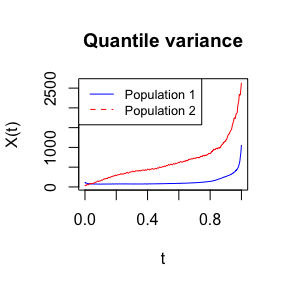The function will plot the quantile mean and the quantile variance of the two populations. The corresponding p-values of the ANOVA test and distributional differences are stored in the following names:

print(htest$energy_pvalue) #>  0.00990099 print(htest$anova_pvalue)
#>  0.0003094763

### Clustering

Call the energy clustering with Wasserstein distance using quantile distributional representations as covariates:

clus = clustering(data1, clusters=3)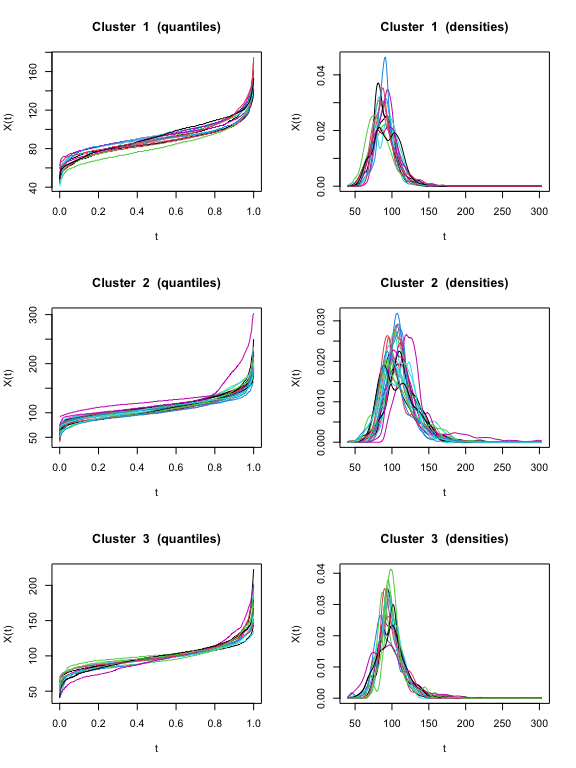The function also plots the clusters of quantiles and densities.

You can also use the previously computed clustering to obtain the clusters of another set of objects calling the following function:

assignments = clustering_prediction(clus, data1$quantiles$data)